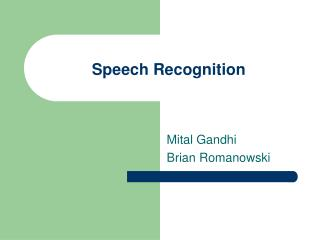DownloadDownload PresentationSpeech Recognition

Speech Recognition

Télécharger la présentationSpeech Recognition

- - - - - - - - - - - - - - - - - - - - - - - - - - - E N D - - - - - - - - - - - - - - - - - - - - - - - - - - -
Presentation Transcript

1. Speech Recognition Mital Gandhi Brian Romanowski

2. Objective - Speech Recognition • Isolated Word Recognition • Portable and Fast

3. System Block Diagram

4. Recognition – Conceptually • Data Acquisition • Training Hidden Markov Models for word set • Recognition & Analysis

5. Theory – Hidden Markov Models • Used to model semi-stationary random processes, like speech • Example: • cat = / k a t /

6. Viterbi-based Recognition • Calculates the log-maximum likelihood of a series of observations given a particular HMM. • “Which model did this set of data most likely come from?” • Saves time by calculating only a subset of possible paths through the HMM network. • At each new frame, only the most likely transition/observation state pairs are used. • Concepts similar to Dynamic Time Warping

7. System Components I Volume Box • Sound Input • Amplifier • Reference Voltage • Resistor network (Voltage Dividers) • Voltage followers • Comparator • Microphone voltage vs. Reference • Output • LED bargraph

8. System Components II Hidden Markov Modeling ToolKit • Data Acquisition • Data Preparation • Parameter Enhancements • Recognition & Analysis

9. System Components II (cont.) HTK: Data Acquisition & Preparation • Data Acquisition • Recording using HSLab • Live audio input using HVite • Data Preparation • External files: dictionary, config, word lists • Initialization of prototype models (HCompV)

10. System Components II (cont.) HTK: Sample External Files • Config • Prototype Model

11. System Components II (cont.) HTK: Training & Recognition • HERest – parameter re-estimation and enhancement tool • Uses information from the energy, delta, & acceleration features in the cepstral domain • HVite for Recognition • Recognition of pre-recorded files or live audio input • A host of external files to support the recognition • Analysis tool HResults to compute accuracy & correctness results

12. System Components II (cont.) HTK: Results & Analysis • HResults • Computes % values for recognition accuracy and correctness • Results Analysis • NREF = percentage of reference labels correctly recognized • Correction does not penalize for insertion errors

13. System Components II (cont.) HTK: Preliminary Results ====================== HTK Results Analysis ====================== Date: Mon Sep 30 16:50:59 2002 Ref : 4word_word.mlf Rec : recout.mlf ------------------------ Overall Results -------------------------- SENT: %Correct=25.00 [H=1, S=3, N=4] WORD: %Corr=25.00, Acc=25.00 [H=9, D=0, S=3, I=0, N=12] ======================

14. System Components II (cont.) HTK: Techniques, Solutions • Input File Specifications • Config • Cepstral mean subtraction, energy enormalization • Prototype model • Number of states per word model • “Optimality” in transition probability assignments (matrix) • Data • “Noise-free” data • As many tokens/samples of each word for training

15. DSP – System Overview • Initialization • Threshold/Recording • MFCC • Viterbi • Output

16. DSP - Matlab • Prototype of all important algorithms • Pre-calculated data • Run-time altering of data (debugging) • Downloading and visualization of data • MFCCs

17. DSP – Recording/Thresholding • Speech Input • Process • Poll A/D for input data (TI-provided code used) • Take only one channel as input • Downsample • Save samples only when signal threshold has been crossed • Lead buffer • Tail buffer • PROBLEMS • Sample transfer modes, single channel selection, threshold values, external microphones • TESTING • Visual and audio inspection in Matlab

18. DSP – MFCC calculation (1) • Thank You to Takuya Ooura for his Public Domain FFT code. • MFCCs provide an uncorrelated and small set of observation vectors for the HMMs • Process: • Remove DC gain • Pre-emphasize • Hamming window • FFT magnitude • Mel-filter bank • DCT • Lifter

19. DSP – MFCC calculation (2) • PROBLEMS: • An incorrectly coded pre-emphasis filter • TESTING: • Graphically compared DSP generated MFCCs to: • Matlab MFCCs -> DSP numerical issues • HTK MFCCs -> reference implementation

20. DSP – Viterbi/Recognition • Uses HTK derived HMMs whose data is contained in a Matlab-generated #include file • PROBLEMS • Numerical concerns • Errors in deriving and coding the formulas.

21. Final Component Results I: HTK • Pre-recorded Files: ====================== HTK Results Analysis ====================== Date: Mon Dec 02 11:37:46 2002 Ref : testwords.mlf Rec : testwordsoutput.mlf ------------------------ Overall Results -------------------------- SENT: %Correct=94.85 [H=92, S=5, N=97] WORD: %Corr=98.28, Acc=98.28 [H=286, D=0, S=5, I=0, N=291] ====================== • Live Audio Input: ~ 83% • DSP MFCC Files: ~ 65 %

22. Final Component Results II: DSP • 95% recognition accuracy over 90 trials • 4 words • Trained speaker • Speaker Independence • Indication of some recognition for non-modeled speakers, but not much • Speech => Decision takes approximately 0.88 seconds

23. Challenges • Speed • Complex project • System integration • Microphone input • Volume Box • HTK • MATLAB & DSP

24. Recommendations • HTK and DSP • Larger training corpus • Multiple Gaussian mixtures • Channel independence • Continuous Recognition • Real-time MFCC transmission from DSP to HTK • DSP • Code style-fixes • Better user interface

25. Thank You • Dan Block – For use of his lab and equipment

26. DSP – MFCC calculation • Thank You to Takuya Ooura for his Public Domain FFT code. • MFCC’s provide an uncorrelated and small set of observation vectors for the HMM’s • Process: • Remove DC gain • Pre-emphasize • Hamming window • FFT magnitude • Mel-filter bank • DCT • Lifter • PROBLEMS: • An incorrectly coded pre-emphasis filter • TESTING: • Graphically compared DSP generated MFCC’s to: • Matlab MFCC’s -> DSP numerical issues • HTK MFCC’s -> reference implementation